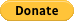# d3-force-magnetic

A natural attraction/repulsion force type for the d3-force simulation engine.

## Usage no npm install needed!

``````<script type="module">
import d3ForceMagnetic from 'https://cdn.skypack.dev/d3-force-magnetic';
</script>``````

# d3.forceMagnetic

An attraction/repulsion force type for the d3-force simulation engine. By default, it follows the inverse-square law (force intensity inversely proportional to the square of the distance), making it suitable for modelling natural occurring forces like gravity, electrostatic or magnetic. This force can be applied system-wide to a group of nodes (using a Barnes-Hut approximation for performance improvement) in which each node affects and is affected by every other node, or alternatively as a collection of specific links pairing two nodes together, with a dedicated intensity.

The intensity of the force between two nodes is determined by the distance between them (`D`), the charge of the opposite node (`C`), the strength of the link (`L`) and the simulation alpha (`A`), using the following formula: `ACL/D^2`.

In the case of a full-mesh group of nodes, the strength of the links is equal for all node pairs, rendering `L` a system constant. When modelling gravity this would be your gravitational constant `G` (and `C` each node's mass), while in an electrostatic system it would represent the Coulomb's law constant `k` (and `C` each node's electrical charge).

Node charges (`C`) can be positive or negative. Positive means that this node will attract other nodes with the specified intensity, while a negative charge represents a repelling force towards other nodes. Keep in mind that, unlike electrical charge, two positive charges do not repel each other, and two opposite charges do not mutually attract each other, a node's charge sign merely represents the effect it has on other nodes. This behavior can however be modified using the `polarity` method to follow the attraction-of-opposites or any other attraction logic.

As example, this force can be used to simulate accretion between particles, or to model orbits of celestial bodies.

This force plugin is also compatible with d3-force-3d and can function in a one, two (default) or three dimensional space.

## Quick start

``````import d3ForceMagnetic from 'd3-force-magnetic';
``````

or

``````d3.forceMagnetic = require('d3-force-magnetic');
``````

or even

``````<script src="//unpkg.com/d3-force-magnetic"></script>
``````

then

``````d3.forceSimulation()
.nodes(<myNodes>)
.force('magnetic', d3.forceMagnetic()
.strength(0.8)
);
``````

## API reference

Method Description Default
links([array]) Getter/setter for the list of links connecting nodes. Setting this value will override the default mode of full-mesh of nodes. Each link should follow the syntax: `{source: <node id>, target: <node id>}`. (full-mesh)
id([fn]) Getter/setter for the node object unique id accessor function, used by links to reference nodes. `node.index`
charge([num or fn]) Getter/setter for the node object charge accessor function (`fn(node)`) or a constant (`num`) for all nodes. A node's charge dictates how other nodes are influenced by it, either by attraction (positive charge) or repulsion (negative charge). 100
strength([num or fn]) Getter/setter for the link object strength accessor function (`fn(link)`) or a constant (`num`) for all node pairs. A link's strength determines how strongly the attractive or repellent force between two nodes is applied to them, or in other words, the capacity of the medium to propagate that mutual force, either by dampening or amplifying. A value of 1 represents unity, while a 0 means no force interaction. 1
polarity([boolean or fn]) Getter/setter for the acceleration polarity function (`fn(charge1, charge2)`) or a constant (`boolean`) for all node pairs. A link acceleration polarity determines whether two given nodes should attract (`true`) or repel (`false`) each other, based on their charges. This can be used f.e. to encode an attraction-of-opposites mode, typical of electrical charges, as `(q1,q2)=>q1*q2<0`. If this method returns `null`, the attraction logic will be left to the assymetrical charge signs of the individual nodes. `null`
distanceWeight([fn]) Getter/setter for the distance relationship function (`fn(distance)`). This method defines how the absolute distance (positive `int`) between two nodes influences the intensity of the attraction force between them. `d=>1/(d*d)` (inverse-square)
theta([number]) Getter/setter for the Barnes-Hut approximation θ threshold value. This parameter is only applicable when using the full-mesh mode. It determines the threshold of how far the node needs to be from a particular quadtree region, relative to the region's width, in order for the approximation to be used, and therefore the accuracy of the force calculation. Longer distances (lower θs) will yield more accurate results, at the cost of performance. 0.9

## Giving BackIf this project has helped you and you'd like to contribute back, you can always buy me a ☕!